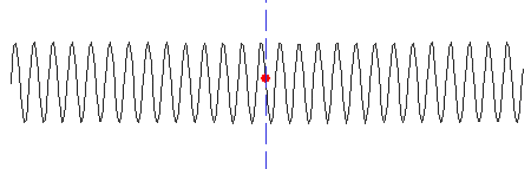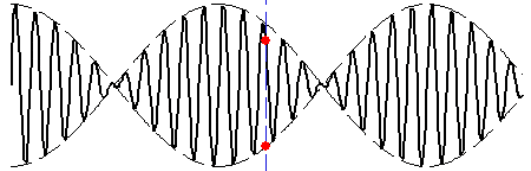## Beats

Beats are caused by the interference between two waves of the same amplitude, travelling with the same wave speed, but having slightly different frequencies, f1 and f2. When the two waves are added at a point in space, a pressure fluctuation is produced whose frequency will be the average, (f1 + f2)/2, of the frequencies of the two original waves.

However, as the two waves will sometimes reinforce each other, and sometimes cancel each other out, we will hear a variation in sound amplitude. This amplitude variation causes the perception of ‘beats’. The frequency of the amplitude variation will be equal to the difference of the two frequencies ((f1f2) if f1 is the higher frequency). This is called the Beat Frequency.

The beat phenomenon is illustrated in the following animation which shows how beats arise due to a small difference in frequency between two sine wave components producing tones respectively at 300 Hz (top curve) and 303 Hz (middle curve). When the two upper red points move in phase, the two lower red points are located close to a maximum amplitude variation of the resulting sound. On the other hand, when the two upper red points move out of phase, the two lower red points are located close to a minimum amplitude variation of the resulting sound.

#### Sinewave 300 Hz#### Sinewave 303 Hz#### Beats